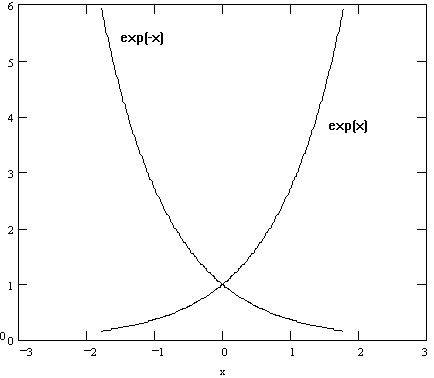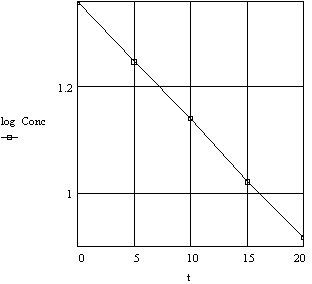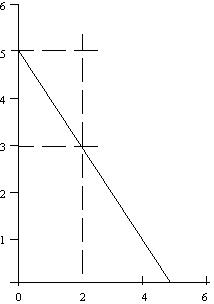Logarithms and Exponential Functions
Logarithms       Log & Exp        Approaches       Problems

One of the basic properties of numbers is that they may be expressed in exponential form. We are all familiar with the representation 1000 = 103 or 0.001 = 10-3. A more general way of stating this property is to say that any number (N) may be expressed as a base (B) raised to a power (x) or
N = Bx

In the examples above the formula the base is 10. Further examples using base 10, base 2, and base e (where e = 2.718...) are given below in Table 1.

 Table 1 Number = 10x Number = 2x Number = ex .01 10-2 1 20 .01 e-4.605 1 100 2 21 1 e0 10 101 4 22 10 e2.303 1000 103 8 23 1000 e6.908 2 10.301 2 e.693 4 10.602 4 e1.386 8 10.903 8 e2.079

The first four entries in the base-10 section look natural as do the entries in the base 2, but few students would immediately guess .301 as the appropriate exponent for 2 = 10x. Further, the natural base e (e = 2.71828..) probably seems at first an illogical base for representing numbers. As will be discussed below, exponential functions of the type y = aebx are very common in describing physical and chemical systems, and a basic understanding of this type of function is necessary.LOGARITHMS

The mathematical statement N = Bx serves as the basis for defining logarithms. The logarithm of a number (N) in base (B) is defined as (x).

logarithm(B)N = x

Two bases, 10 and e, are in common usage. The shorthand representations are:

base 10 logarithm10 N = log10 N = log N
and
base e ("Natural" logarithms) logarithme N = ln N
Thus, the logarithm of a number is simply the power to which the base must be raised to give the number. Table 2 shows the log and ln of the numbers in Table 1.

 Table 2 Number log ln .0100 -2.000 -4.605 1.00 0 0 10.0 1.000 2.303 1000 3.000 6.908 2.00 0.301 0.693 4.00 0.602 1.386 8.00 0.903 2.079

NOTE:
Only the numbers to the right of the decimal point in a logarithm are significant figures. The number to the left of the decimal point simply, in effect, tells us where the decimal point is and is not considered a significant figure.

There are a few basic rules for handling logarithms. Examples are given in base 10 but the rules are applicable to any base.

Rule 1:         log (a x b) = log a + log b
Examples:

log (2000) = log (2 x 1000) = log 2 + log 1000 = .301 + 3 = 3.301
log (.004) = log (4 x .001) = log 4 + log .001  = .602 - 3 = -2.398
Rule 2:         log (a/b) = log a - log b
Examples:
log (2/4) = log 2 - log 4 = .301 - .602 = -.301
log (2/.4) = log 2 - log .4 = log 2 - [log (4 x .1)] = .301 - [.602 - 1] = .699
Rule 3:         log (a)b = b log a
Examples:
log (4)3 = 3 log 4 = 3 x .602 = 1.806
log (8)1/2 = 1/2 log 8 = 1/2 x .903 = .452
Rule 4:         log (10)x = x
Examples:
log 10-8 = -8
ln e4 = 4
If one has the logarithm of a number and wishes to find the number, one simply raises the base to the power of the logarithm. This is called taking the antilogarithm.

Examples:

antilog .301 = 10.301 = 2
antilog 1.806 = 101.806 = 64
antilog -2.398 = 10-2.398 = .004
antiln 2.303 = e2.303 = 10
antiln 1.386 = e1.386 = 4
Note: On most calculators, antilogarithms may be taken by
• INV + log or INV + ln

• or
• 10x or ex

• or
• yx where y = BASE.
While logarithms are interesting and useful in their own right, they have greatest applicability for us in dealing with exponential functions.LOGARITHMIC AND EXPONENTIAL FUNCTIONS

One of the very common functional relationships appearing from experimental observations is that of an exponential increase or decrease. This takes the form of an expression as

y = ex and y = e-x

In the more general form this appears as

y = eax and y = e-ax

where a groups together any quantities that can be considered to be constant during the variation of x and y. When graphed, these functions appear as seen in Figure 1 (note that when x = 0, y becomes = 1). Base e logarithms can be converted to base 10 logarithms.

Figure 1. Exponential FunctionsSince it is very difficult to obtain the exact form of the coefficient a from graphs such as these, it becomes more convenient to apply logarithms to functions in this form. Thus,
y = eax becomes ln y = ax; y = 10a'x becomes log y = a'x

or 2.3 log y = ax.        [Note that a' = a/2.3 ]

and a plot of ln (or log) y versus x will then give a straight line whose slope will be "a." The same considerations apply to these expressions when they are straight lines as to any straight line.

In a simple case, consider the data from Table 3 for the decomposition of hydrogen peroxide. Two ways in which these data can be graphed are shown below: (a) concentration against time on rectangular coordinates, (b) log concentration against time on rectangular coordinates. These are illustrated in Figures 2a - 2b.

 Table 3 Decomposition of Hydrogen Peroxide Time Concentration Log Concentration 0 22.8 1.358 5 17.6 1.245 10 13.8 1.140 15 10.5 1.021 20 8.25 0.916

Figure 2a. Decomposition of Hydrogen Peroxide
Plot of Concentration vs TimeFigure 2b. Decomposition of Hydrogen Peroxide
Plot of log Concentration vs TimeWhether one uses the logarithmic or exponential form for such relationships depends on what is to be determined. Mathematically the exponential form has certain advantages while graphically the logarithmic form is more informative. Any functional relationship in this form has recognizable characteristics that are more obvious when one more symbol is included in the general expression. Thus,

y = yoe-ax

where yo is a constant determined by the fact that when x = 0, the value of e-ax = 1 and under these conditions, y = yo. The following examples illustrate the general characteristics of this type of function. The "a" is a specific constant in each example.

1. The decrease in the concentration of reacting material c per unit time t is proportional to the concentration and is expressed as

2.             c = coe-at
where co is the concentration at time t = 0 (at the beginning of the experiment).
3. The decrease in the intensity of the incident light, I, is proportional to the depth, l, of the absorbing material and is expressed as

4.         I = Ioe-al
where Io is the intensity of the incident radiation at l = 0, before it passes through the absorbing material.
5. The pressure of the atmosphere P decreases at a rate proportional to the altitude h and is expressed as

6.         P = Poe-ah
where Po is the pressure at h = 0, sea level.General Approach to Generating Equation from Data
1. Plot the given numerical values for x and y on a piece of graph paper using y as the vertical axis (ordinate) and x as the horizontal axis (abscissa). If a graphing program for a microcomputer is available, it provides a convenient way go examine a graph without resorting to a paper copy. The experimentally independent value is generally put on the x axis, and the experimentally measured, dependent, variable on the y axis.
2. If the resulting plot shows a straight line, the equation for the original numerical values is easily determined since the equation of a straight line is

3.         y = mx + b
where m = slope and b = intercept of line with y axis (at x=0).
Figure A. Positive slope
slope = m = rise/run = 2/3Figure B. Negative slope
slope = m = rise/run = -2/2 = -1Calculate or read off the values for m and b, then insert the m and b values into the equation y = mx + b. The result should be the equation you're looking for. To double check your work, try some of the original given values for x in your equation and see if y comes out as expected. The equation of the line in Fig. A is y = 2x/3 + 5/3 and for Fig. B is y = -x + 5 = 5 - x.

1. If the plot resulting from step I is not a straight line, you are not particularly likely to happen on the equation by inspection. I.e. the fact that if x = 3, y = 9, (a single point) does not imply that the equation is y = x2 (holds for all points). One method is to convert the original data to a straight line. In this course we will encounter several relationships of the form

2.         y = ae-b/T
where y is some property of the system, a and b are constants and T is the temperature in Kelvins. These functions are all linearized by taking the natural logarithym of the expression.

ln y = ln a - b/T. A plot of ln y vs 1/T will give a straight line with slope -b and intercept ln a.Problems
Ans 1.    Plot the following data in a graph of density versus temperature. Determine the relationship between density and temperature from this graph. Write a mathematical expression for this relationship, and from the graph evaluate all constants.

 Density Temperature (g/mL) (degrees C) 13.60 0 13.55 20.0 13.50 40.0 13.45 60.0 13.40 80.0 13.25 100.0
Ans 2.    Complete the following table.

 Number log ln 4.02 x 10-6 6.02 x 1023 5.01 0.01555 2.86 -3.08 184.301 -5.634 9.825 1.063
Ans 3.     For each of the following equations, indicate what type of scale should be used on the y axis and the x axis to obtain a linear plot. Determine the intercept and slope. Note that more than one approach is often possible.
(a) y = 3x2 + 4
(b) y = 3e2x
(c) y = 6e-4x
(d) y = 3e4/x
(e) y = 2e-8/x